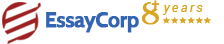WhatsApp
Struggling With Assignments?

We are here to help.

Chat with our expert writers on WhatsApp

for any kind of assignment queries.

Click to chat!
Struggling with Assignments? WhatsApp Us#### Quesion :

Determine the orbit of an object that you have observed. You will determine the object state vector at a time of the first observation and create a simulations of the orbital trajectory.

#### Solution :

The steps followed to solve the problems are as follows:

1. Determine the UTC (Coordinated Universal Time) for the three observations to calculate the Local Sidereal Time for each observation. Nairobi is located at UTC + 3 so the time in UTC is the time given – 3.
2. Calculate the Local Sidereal Time for each observation
Date  Local Time*  Azimuth Angle (deg.)  Elevation Angle (deg.)  Range (km)  Local Sidereal Time  Universal Time
17-05-20  12:06 AM  272.4822  21.9305  39364  229.322  21:06
17-05-20  1:00 AM  272.4401  22.0888  39348  242.859  22:00
17-05-20  1:30 AM  272.3864  22.5174  39307  250.38  22:30
3. Calculate the three geocentric position vectors corresponding to the three sets of azimuth, elevation and slant range data at each time given the earth observation location:
1. Using the altitude H, latitude φ and local sidereal time θ of the site, calculate its geocentric position vector R from the following equation:2. Calculate the topocentric declination δ using the following equation:3. Calculate the topocentric right ascension α using the following equations:4. Calculate the direction cosine unit vector using the following equation:1. Calculate the geocentric position vector r using the following equation:The results obtained by MATLAB were as the following:
4. Applying Gibbs’ Method to the three obtained position vectors to get the velocity vector corresponding to the second position vector: - The result was as following: We take the initial r and v to be r2 and v2:
5. Knowing r and v the 6 COE could be obtained easily using the below flow graph  and the results were:Since the eccentricity is between 0 and 1, the orbit is elliptical.

HIRE EXPERT
Email ID *
Subject
Pages